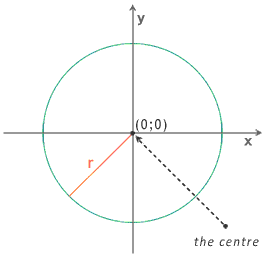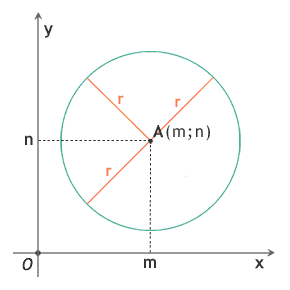# What Is the Circumference of a Circle

Do you want to know what is the circumference of a circle? The circumference of a circle is a geometric figure. You can get it in the geometric plane. Here there is the canonical equation of the circumference with the centre in the point (0;0) and with radius r:

x2 + y2 = r2and another one, with the centre in the point (m;n):

(x - m)2 + (y - n)2 = r2